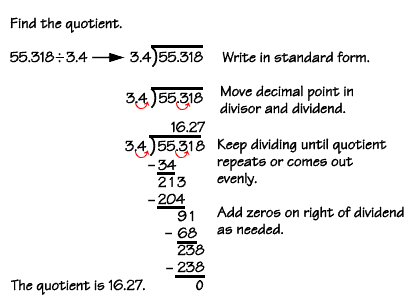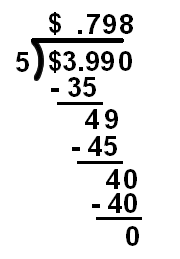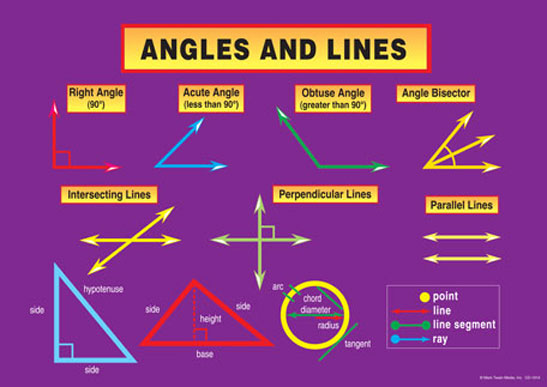### Mr. Cairns' 6th Grade Online Math Academy

#### Math News:

Welcome to Mr. Cairns'

6th Grade Math Academy!

Challenge Day Champs Again!
Looking for something to do

Hi!.  You remember all those extra worksheets that you were asking about for extra practice?
Well, I HAVE A SOLUTION!
INTRODUCING MATH-AIDS!
Create just about any math worksheet you can think of!

Distributive Property

The Distributive Property is easy to remember, if you recall that "multiplication distributes over addition". Formally, they write this property as "a(b + c) = ab + ac". In numbers, this means, that 2(3 + 4) = 2×3 + 2×4. Any time they refer in a problem to using the Distributive Property, they want you to take something through the parentheses (or factor something out); any time a computation depends on multiplying through a parentheses (or factoring something out), they want you to say that the computation used the Distributive Property.

• Why is the following true? 2(x + y) = 2x + 2y

Since they distributed through the parentheses, this is true by the Distributive Property.

• Use the Distributive Property to rearrange: 4x – 8

The Distributive Property either takes something through a parentheses or else factors something out. Since there aren't any parentheses to go into, you must need to factor out of. Then the answer is "By the Distributive Property, 4x – 8 = 4(x – 2)"

"But wait!" you say. "The Distributive Property says multiplication distributes over addition, notsubtraction!  What gives?" You make a good point. This is one of those times when it's best to be flexible. You can either view the contents of the parentheses as the subtraction of a positive number ("x – 2") or else as the addition of a negative number ("x + (–2)"). In the latter case, it's easy to see that the Distributive Property applies, because you're still adding; you're just adding a negative.

The other two properties come in two versions each: one for addition and the other for multiplication. (Note that the Distributive Property refers to both addition and multiplication, too, but to both within just one rule.)

Associative Property

The word "associative" comes from "associate" or "group";the Associative Property is the rule that refers to grouping. For addition, the rule is "a + (b + c) = (a + b) + c"; in numbers, this means
2 + (3 + 4) = (2 + 3) + 4. For multiplication, the rule is "a(bc) = (ab)c"; in numbers, this means2(3×4) = (2×3)4. Any time they refer to the Associative Property, they want you to regroup things; any time a computation depends on things being regrouped, they want you to say that the computation uses the Associative Property.   Copyright © Elizabeth Stapel 2000-2011 All Rights Reserved

• Rearrange, using the Associative Property: 2(3x)

They want you to regroup things, not simplify things. In other words, they do not want you to say "6x". They want to see the following regrouping: (2×3)x

• Simplify 2(3x), and justify your steps.

In this case, they do want you to simplify, but you have to tell why it's okay to do... just exactly what you've always done. Here's how this works:

 2(3x) original (given) statement (2×3)x by the Associative Property 6x simplification (2×3 = 6)
• Why is it true that 2(3x) = (2×3)x?

Since all they did was regroup things, this is true by the Associative Property.

Commutative Property

The word "commutative" comes from "commute" or "move around", so the Commutative Property is the one that refers to moving stuff around. For addition, the rule is "a + b = b + a"; in numbers, this means 2 + 3 = 3 + 2. For multiplication, the rule is "ab = ba"; in numbers, this means 2×3 = 3×2.Any time they refer to the Commutative Property, they want you to move stuff around; any time a computation depends on moving stuff around, they want you to say that the computation uses the Commutative Property.

• Use the Commutative Property to restate "3×4×x" in at least two ways.

They want you to move stuff around, not simplify. In other words, the answer is not "12x"; the answer is any two of the following:

4 × 3 × x, 4 × × 3, 3 × × 4, × 3 × 4, and × 4 × 3

• Why is it true that 3(4x) = (4x)(3)?

Since all they did was move stuff around (they didn't regroup), this is true by the Commutative Property.

Worked examples

• Simplify 3a – 5b + 7a. Justify your steps.

I'm going to do the exact same algebra I've always done, but now I have to give the name of the property that says its okay for me to take each step. The answer looks like this:

 3a – 5b + 7a original (given) statement 3a + 7a – 5b Commutative Property (3a + 7a) – 5b Associative Property a(3 + 7) – 5b Distributive Property a(10) – 5b simplification (3 + 7 = 10) 10a – 5b Commutative Property

The only fiddly part was moving the "– 5b" from the middle of the expression (in the first line of the table above) to the end of the expression (in the second line). If you need help keeping your negativesstraight, convert the "– 5b" to "+ (–5b)". Just don't lose that minus sign!

• Simplify 23 + 5x + 7y – x – y – 27.   Justify your steps.

•  23 + 5x + 7y – x – y – 27 original (given) statement 23 – 27 + 5x – x + 7y – y Commutative Property (23 – 27) + (5x – x) + (7y – y) Associative Property (–4) + (5x – x) + (7y – y) simplification (23 – 27 = –4) (–4) + x(5 – 1) + y(7 – 1) Distributive Property –4 + x(4) + y(6) simplification –4 + 4x + 6y Commutative Property
• Simplify 3(x + 2) – 4x.   Justify your steps.

•  3(x + 2) – 4x original (given) statement 3x + 3×2 – 4x Distributive Property 3x + 6 – 4x simplification (3×2 = 6) 3x – 4x + 6 Commutative Property (3x – 4x) + 6 Associative Property x(3 – 4) + 6 Distributive Property x(–1) + 6 simplification (3 – 4 = –1) –x + 6 Commutative Property
• Why is it true that 3(4 + x) = 3(x + 4)?

All they did was move stuff around: Commutative Property

• Why is 3(4x) = (3×4)x?

All they did was regroup: Associative Property

• Why is 12 – 3x = 3(4 – x)?

They factored: Distributive PropertyWHO IS GOING TO WIN THE KHAN ACADEMY PIZZA PARTY?!Resilience...Do you have it?!OF MATH VIDEO LESSONS AND HELP SITES!Reciprocal!!!!!!ONLINE MATH BOOK!

Lab Students!  Behold!  XTRA MATH!

PRE ALGEBRA: Here are some great videos for you also!

You want great searchable lessons?...YOU GOT IT!

Click Here for Sum Dog!

Dividing by DecimalsDividing Decimals by Whole NumbersVIDEO LESSONS AND HElP SITES
Lesson by Lesson for 2014 - 2015
Ordered Pairs - Interactive Site: Click Here

Last year's crop of lessons that will apply later this year!
Understanding Whole Numbers Click Here
U.S. Customary Measurement: Click Here
The Metric System: Click Here
All About Geometry: Click Here
Multiplying Fractions: Click Here
Finding the Average: Click Here
Mode, Median, and Range Examples:  Click Here
Eqivalent Fractions: Click Here
Fraction Word Problems: Click Here
Equivalent Fractions Interactive Quiz: Click Here
Fractions by Zeebo(King of the Fractions!):Click Here
Geometry Worksheets: Click Here
Long Division Videos Click Here
Fact Families Game: Click Here
Multiplication.com Click Here
Adding with Regrouping Click Here
Fractions Help: Click Here
Multiplying by 100's: Click Here
Division With Remainders: Click Here
Printable Flash Cards: Click Here
Math Cats...Really cool math games! Click Here
Telling Time Game: Click Here
Decimals - Tenths and Hundredths: Click Here
Subtraction Across Zeros Video: Click Here
Prime and Composite Battleship Game: Click Here
Baseball Math: Click Here
Cool Math 4 Kids: Click Here
Elapsed Time Matching Game: Click Here
The Best Math Practice Page on the Net!: Click Here
2 Digit X 2 Digit Multiplication: Click Here
Place Value Game:
Place Value: Click Here
Bar Graphs: Click Here
Line Graphs: Click Here
Line Plots: Click Here
Basic Addition: Click Here
Basic Subtraction: Click Here

Getting Ready for Common Core in 6th Grade.  Here is what you need to know!

 M06.A-N The Number System ASSESSMENT ANCHORM06.A-N.1 Apply and extend previous understandings of multiplication and division to divide fractions by fractions. M06.A-N.1.1 Solve real-world and mathematical problems involving division of fractions. M06.A-N.1.1.1 - Interpret and compute quotients of fractions (including mixed numbers), and solve word problems involving division of fractions by fractions.Example 1: Given a story context for (2/3) ÷ (3/4), explain that (2/3) ÷ (3/4) = 8/9 because 3/4 of 8/9 is 2/3. (In general, (a/b) ÷ (c/d) = (a/b) × (d/c) = ad/bc.)Example 2: How wide is a rectangular strip of land with length 3/4 mi and area 1/2 square mi?Example 3: How many 2 1/4-foot pieces can be cut from a 15 1/2-foot board? Division of Fractions ASSESSMENT ANCHORM06.A-N.2 Compute with multi-digit numbers and find common factors and multiples. M06.A-N.2.1 Compute with multi-digit numbers using the four arithmetic operations with or without a calculator. M06.A-N.2.1.1 - Solve problems involving operations (+, -, ×, and ÷) with whole numbers, decimals (through thousandths), straight computation, or word problems. Arithmetic with DecimalsArithmetic with Whole Numbers M06.A-N.2.2 Apply number theory concepts (specifically, factors and multiples). M06.A-N.2.2.1 - Find the greatest common factor of two whole numbers less than or equal to 100 and the least common multiple of two whole numbers less than or equal to 12. Number Theory M06.A-N.2.2.2 - Apply the distributive property to express a sum of two whole numbers, 1 through 100, with a common factor as a multiple of a sum of two whole numbers with no common factor.Example: Express 36 + 8 as 4(9 + 2). Number Theory ASSESSMENT ANCHORM06.A-N.3 Apply and extend previous understandings of numbers to the system of rational numbers. M06.A-N.3.1 Understand that positive and negative numbers are used together to describe quantities having opposite directions or values and locations on the number line and coordinate plane. M06.A-N.3.1.1 - Represent quantities in real-world contexts using positive and negative numbers, explaining the meaning of 0 in each situation (e.g., temperature above/below zero, elevation above/below sea level, credits/debits, positive/negative electric charge). Negative and Positive Numbers M06.A-N.3.1.2 - Determine the opposite of a number and recognize that the opposite of the opposite of a number is the number itself (e.g., -(-3) = 3; 0 is its own opposite). Number Lines M06.A-N.3.1.3 - Locate and plot integers and other rational numbers on a horizontal or vertical number line; locate and plot pairs of integers and other rational numbers on a coordinate plane. Coordinate PlanesNumber Lines M06.A-N.3.2 Understand ordering and absolute value of rational numbers. M06.A-N.3.2.1 - Write, interpret, and explain statements of order for rational numbers in real-world contexts.Example: Write -3°C > -7°C to express the fact that -3°C is warmer than -7°C. Negative and Positive Numbers M06.A-N.3.2.2 - Interpret the absolute value of a rational number as its distance from 0 on the number line and as a magnitude for a positive or negative quantity in a real-world situation.Example: For an account balance of -30 dollars, write |-30| = 30 to describe the size of the debt in dollars, and recognize that an account balance less than -30 dollars represents a debt greater than 30 dollars. Absolute Value M06.A-N.3.2.3 - Solve real-world and mathematical problems by plotting points in all four quadrants of the coordinate plane. Include use of coordinates and absolute value to find distances between points with the same first coordinate or the same second coordinate. Coordinate Geometry M06.A-R Ratios and Proportional Relationships ASSESSMENT ANCHORM06.A-R.1 Understand ratio concepts and use ratio reasoning to solve problems. M06.A-R.1.1 Represent and/or solve real-world and mathematical problems using rates, ratios, and/or percents. M06.A-R.1.1.1 - Use ratio language notation (such as 3 to 4, 3:4, 3/4) to describe a ratio relationship between two quantities.Example 1: "The ratio of girls to boys in a math class is 2:3 because for every 2 girls there are 3 boys."Example 2: "For every five votes candidate A received, candidate B received four votes." Ratios and Ratio Language M06.A-R.1.1.2 - Find the unit rate a/b associated with a ratio a:b (with b0) and use rate language in the context of a ratio relationship. Example 1: "This recipe has a ratio of 3 cups of flour to 4 cups of sugar, so there is 3/4 cup of flour for each cup of sugar."Example 2: "We paid \$75 for 15 hamburgers, which is a rate of \$5 per hamburger." Unit Rates M06.A-R.1.1.3 - Construct tables of equivalent ratios relating quantities with whole-number measurements, find missing values in the tables, and/or plot the pairs of values on the coordinate plane. Use tables to compare ratios. Units of Measurement M06.A-R.1.1.4 - Solve unit rate problems including those involving unit pricing and constant speed.Example: If it took 7 hours to mow 4 lawns, than at that rate, how many lawns could be mowed in 35 hours? At what rate were lawns being mowed? Unit Rates M06.A-R.1.1.5 - Find a percent of a quantity as a rate per 100 (e.g., 30% of a quantity means 30/100 times the quantity); solve problems involving finding the whole, given a part and the percentage. Percents M06.B-E Expressions and Equations ASSESSMENT ANCHORM06.B-E.1 Apply and extend previous understandings of arithmetic to numerical and algebraic expressions. M06.B-E.1.1 Identify, write, and evaluate numerical and algebraic expressions. M06.B-E.1.1.1 - Write and evaluate numerical expressions involving whole-number exponents. Evaluate ExpressionsWrite Expressions M06.B-E.1.1.2 - Write algebraic expressions from verbal descriptions.Example: Express the description "five less than twice a number" as 2y - 5. Write Expressions M06.B-E.1.1.3 - Identify parts of an expression using mathematical terms (e.g., sum, term, product, factor, quotient, coefficient, quantity).Example: Describe the expression 2(8 + 7) as a product of two factors. Write Expressions M06.B-E.1.1.4 - Evaluate expressions at specific values of their variables, including expressions that arise from formulas used in real-world problems.Example: Evaluate the expression b2 - 5 when b = 4. Evaluate Expressions M06.B-E.1.1.5 - Apply the properties of operations to generate equivalent expressions.Example 1: Apply the distributive property to the expression 3(2 + x) to produce the equivalent expression 6 + 3x.Example 2: Apply the distributive property to the expression 24x + 18y to produce the equivalent expression 6(4x + 3y).Example 3: Apply properties of operations to y + y + y to produce the equivalent expression 3y. Equivalent Expressions ASSESSMENT ANCHORM06.B-E.2 Interpret and solve one-variable equations and inequalities. M06.B-E.2.1 Create, solve, and interpret one-variable equations or inequalities in real-world and mathematical problems. M06.B-E.2.1.1 - Use substitution to determine whether a given number in a specified set makes an equation or inequality true. Solve Equations and Inequalities M06.B-E.2.1.2 - Write algebraic expressions to represent real-world or mathematical problems. Symbolize Problem Situations M06.B-E.2.1.3 - Solve real-world and mathematical problems by writing and solving equations in the form x + p = q and px = q for cases in which p, q, and x are all non-negative rational numbers. Number Sentences M06.B-E.2.1.4 - Write an inequality of the form x > c or x < c to represent a constraint or condition in a real-world or mathematical problem and/or represent solutions of such inequalities on number lines. Number Sentences ASSESSMENT ANCHORM06.B-E.3 Represent and analyze quantitative relationships between dependent and independent variables. M06.B-E.3.1 Use variables to represent two quantities in a real-world problem that change in relationship to one another. M06.B-E.3.1.1 - Write an equation to express the relationship between the dependent and independent variables.Example: In a problem involving motion at a constant speed of 65 units, write the equation d = 65t to represent the relationship between distance and time. Quantitative Relationships M06.B-E.3.1.2 - Analyze the relationship between the dependent and independent variables using graphs and tables and/or relate these to an equation. Quantitative Relationships M06.C-G Geometry ASSESSMENT ANCHORM06.C-G.1 Solve real-world and mathematical problems involving area, surface area, and volume. M06.C-G.1.1 Find area, surface area, and volume by applying formulas and using various strategies. M06.C-G.1.1.1 - Determine the area of triangles and special quadrilaterals (i.e., square, rectangle, parallelogram, rhombus, and trapezoid). Formulas will be provided. Area M06.C-G.1.1.2 - Determine the area of irregular or compound polygons.Example: Find the area of a room in the shape of an irregular polygon by composing and/or decomposing. Area M06.C-G.1.1.3 - Determine the volume of right rectangular prisms with fractional edge lengths.Formulas will be provided. Surface Area and Volume M06.C-G.1.1.4 - Given coordinates for the vertices of a polygon in the plane, use the coordinates to find side lengths and area of the polygon (limited to triangles and special quadrilaterals). Formulas will be provided. Coordinate Geometry M06.C-G.1.1.5 - Represent three-dimensional figures using nets made of rectangles and triangles. Surface Area and Volume M06.C-G.1.1.6 - Determine the surface area of triangular and rectangular prisms (including cubes). Formulas will be provided. Surface Area and Volume M06.D-S Statistics and Probability ASSESSMENT ANCHORM06.D-S.1 Demonstrate understanding of statistical variability by summarizing and describing distributions. M06.D-S.1.1 Display, analyze, and summarize numerical data sets in relation to their context. M06.D-S.1.1.1 - Display numerical data in plots on a number line, including line plots, histograms, and box-and-whisker plots. Graphing and Interpreting Data M06.D-S.1.1.2 - Determine quantitative measures of center (e.g., median, mean, mode) and variability (e.g., range, interquartile range, mean absolute deviation). Graphing and Interpreting DataStatistical Analysis M06.D-S.1.1.3 - Describe any overall pattern and any deviations from the overall pattern with reference to the context in which the data were gathered. Graphing and Interpreting DataStatistical Analysis M06.D-S.1.1.4 - Relate the choice of measures of center and variability to the shape of the data distribution and the context in which the data were gathered. Statistical Analysis

Class Description

Each Chapter in our Math book is divided up into 4-18 sections. We will be covering all of the sections in our curriculum only, therefore some of the sections that are not included in our curriculum may not be taught. Also, we may jump around from chapter to chapter to allow for better coverage of the materials, so do not be alarmed. Each unit will have 1-2 quizzes and 1 final test.

Contacting Me

If you have questions about anything during the year, please don’t hesitate to e- mail, write a note, or call me at the intermediate school. E-MAIL IS THE MOST EFFICIENT WAY TO REACH ME. I will get back to you within 24 hours. The number is 412-429-2220. It is a pleasure having your child in class. Thank you, in advance, for your support throughout the year.

# Homework Tracker

The students will be required to place their assignments for each night in a homework tracker. Each night the tracker should come home with them. Please verify that your child has completed all of the work recorded in the tracker by initialing it daily in the space provided. These trackers were provided by our PTG.  Also, all homework will be posted on the CVMS 6th grade Red Team page.

Just had to put this photo up there!

Comments